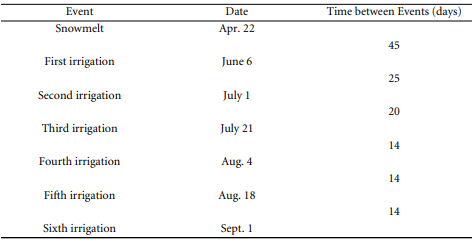## Determine the peak discharge due to deep percolation and upstream slope.

For the parallel pipe drains of Problem 17.5, the length of drain is 7230 ft. The surface gradient is 0.5%. The water table is generally parallel to the ground surface. Determine the peak discharge due to deep percolation and upstream slope. Also design the drain. n = 0.011.

Problem 17.5

For an agricultural drainage system, design the drains (determine the drain spacing) for the following conditions:

1. Depth from surface to impervious layer = 30 ft

2. Depth of drains from surface = 8 ft

3. Root zone or water-table requirement = 4 ft below the surface

4. Uniform hydraulic conductivity = 10 ft/day

5. Water application is as follows:6. Each irrigation application, as well as spring snowmelt, contributed to a deep percolation of 1 in. (Adapted from the Bureau of Reclamation, 1984.)

### Explain why attenuation is not a big problem in PET.

Consider a 2-D object consisting of two triangle compartments, as shown in Figure P9.4. Suppose a solution containing a 511 KeV gamma ray emitting radionuclide with concentration f = 0.5….

### Give the mean and the variance of the reconstructed image, mean[ˆ f(x, y)] and var[ˆ f(x, y)].

Ignoring the inverse square law and attenuation, an approximate reconstruction for SPECT imaging is given by where c˜() =  {||W()} and W() is a rectangular windowing filter that cuts off at = 0…..

### Find the numerical responses in each to an event in crystal C(4, 6).

Suppose a PET detector comprises four square PMTs (arranged as a 2 by 2 matrix) and a single BGO crystal with slits made in such a way that it is….